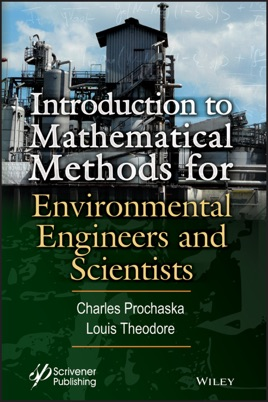• \$189.99

## Publisher Description

The material in this book attempts to address mathematical calculations common to both the environmental science and engineering professionals. The book provides the reader with nearly 100 solved illustrative examples. The interrelationship between both theory and applications is emphasized in nearly all of the 35 chapters. One key feature of this book is that the solutions to the problems are presented in a stand-alone manner. Throughout the book, the illustrative examples are laid out in such a way as to develop the reader’s technical understanding of the subject in question, with more difficult examples located at or near the end of each set.

In presenting the text material, the authors have stressed the pragmatic approach in the application of mathematical tools to assist the reader in grasping the role of mathematical skills in environmental problem-solving situations. The book is divided up into five (V) parts: Introduction Analytical Analysis Numerical Analysis Statistical Analysis Optimization